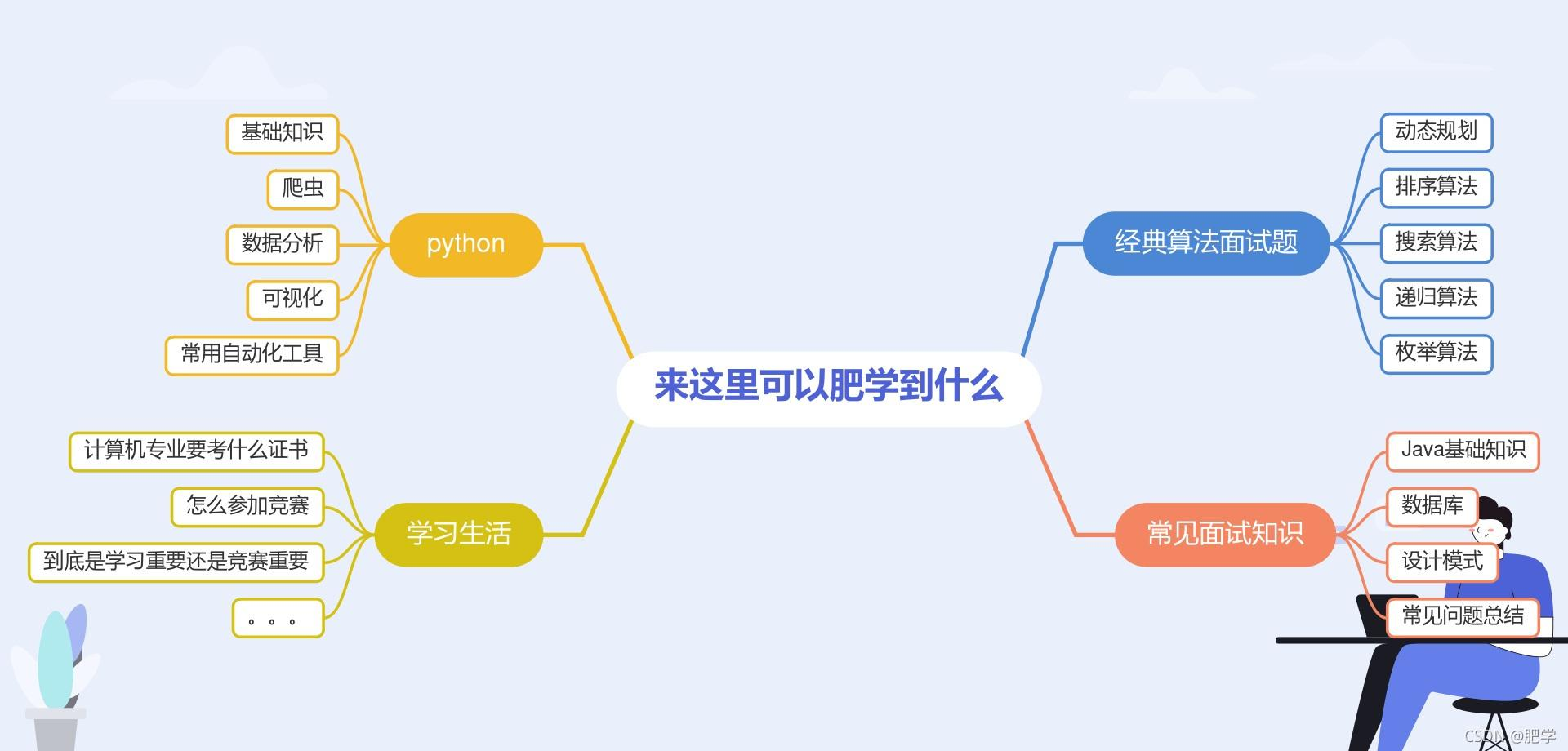衝刺大廠每日演算法&面試題，動態規劃21天——第十八天

highlight: a11y-dark theme: mk-cute

「這是我參與11月更文挑戰的第16天，活動詳情檢視：2021最後一次更文挑戰

導讀21天動態規劃入門

```java 示例 1：

```java 示例 2：

```java 示例 3：

```java class Solution { public int lengthOfLIS(int[] nums) { if (nums.length == 0) { return 0; } int[] dp = new int[nums.length]; dp = 1; int maxans = 1; for (int i = 1; i < nums.length; i++) { dp[i] = 1; for (int j = 0; j < i; j++) { if (nums[i] > nums[j]) { dp[i] = Math.max(dp[i], dp[j] + 1); } } maxans = Math.max(maxans, dp[i]); } return maxans; } }

```

面試題

public class node { public int val; public node left;//左孩子 public node right;//右孩子 public node() {

}
public node(int val,node left,node right) {
this.val=val;
this.left=left;
this.right=right;
}
public node(int val, node left) {
super();
this.val = val;
this.left = left;
}

}

```

```java public class kTierNums { //求第k層節點的個數 public int kNums(node root,int k) { if(root==null||k<1)return 0; if(k==1)return 1; int leftNum=kNums(root.left,k-1); int rightNum=kNums(root.right,k-1); return leftNum+rightNum; }

} ``` 問：怎麼判斷是否為平衡二叉樹

```java package tree;

public class AVL { //判斷是不是平衡二叉樹 public int BalancedTree(node root) { if(root==null)return 0; int left=BalancedTree(root.left); int right=BalancedTree(root.right); if(left==-1||right==-1||Math.abs(left-right)>1) { return -1; } return Math.max(left, right)+1; } public boolean isBalanced(node root) { return BalancedTree(root)!=1; } }

```